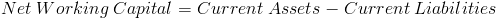# Net Working CapitalThe formula for net working capital (NWC), sometimes referred to as simply working capital, is used to determine the availability of a company's liquid assets by subtracting its current liabilities.

Current Assets are the assets that are available within 12 months. Current Liabilities are the liabilities that are due within 12 months.

## Use of Net Working Capital Formula

Net working capital is used in various other financial formulas that deal with cash flows. Examples of these formulas include the free cash flow to equity formula and free cash flow to firm formula.

In the formula for free cash flow to equity, the change in net working capital is subtracted. An increase in net working capital is considered a negative cash flow and not available for equity. In other words, an increasing requirement for capital for short term operations in the company is not available to equity.

The variables of the net working capital formula are the same as those used in the current ratio. The current ratio formula instead divides current assets by current liabilities. And such, a company with a current ratio of greater than 1 will have positive net working capital. These formulas, along with others, are referred to as liquidity ratios as they are measures of a company's ability to meet its short term obligations.

New to Finance?